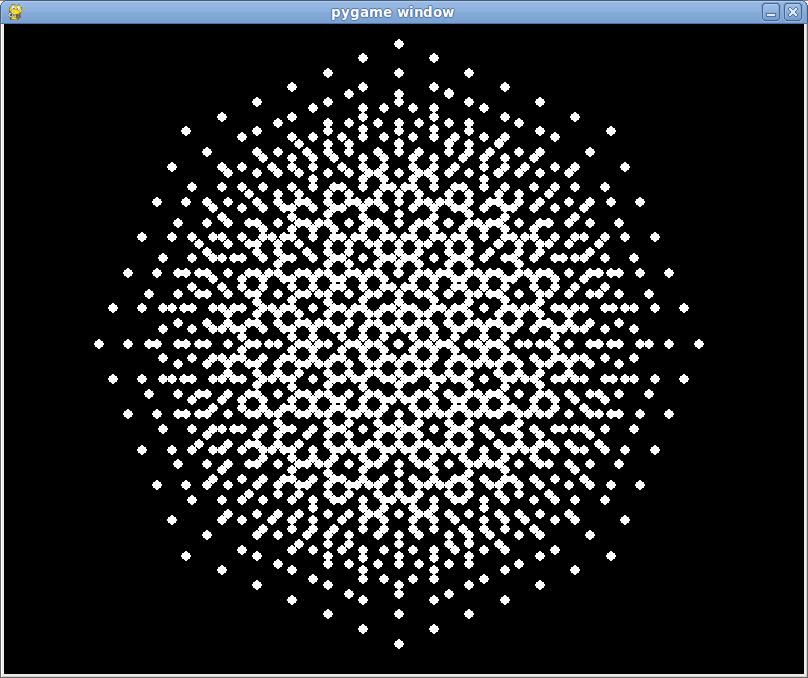# Pretty fractal

I was experimenting with a pseudo-many-worlds quantum physics idea, and this screenshot came of it:Beautiful, isn't it? Here's the source if you want to play around, it's optimized as much as I know how without switching to C, any suggestions would be welcome:

import pygame
from pygame.locals import *
from math import sin, cos, pi

pygame.init()
screen = pygame.display.set_mode((800,650), HWSURFACE|DOUBLEBUF)
clock = pygame.time.Clock()

base = pygame.Surface((10,10))
pygame.draw.circle(base, (255,255,255), (5,5), 5)
base.set_colorkey((0,0,0))

next_circs = [(395,320)]

keep_going = 1
poses = {}
angs = range(9)
off = 50
while keep_going:
clock.tick(1)
for event in pygame.event.get():
if event.type == QUIT or (event.type == KEYDOWN and event.key == K_ESCAPE):
keep_going = 0

print len(next_circs)
next_circs_h = []
for c in next_circs:
poses[c] = 0
screen.blit(base, (c - 5, c - 5))

for i in angs:
topleft = c + off*cos(i*pi/4), c + off*sin(i*pi/4)
if not poses.has_key(topleft):
next_circs_h.append(topleft)
next_circs = next_circs_h
pygame.display.flip()


Note I also tried two memoization techniques but the raw sin and cos appear to be faster anyway:


# first attempt
class memoize:
def __init__(self, fn):
self.mems = {}
self.fn = fn

def __call__(self, x):
if self.mems.has_key(x):
return self.mems[x]
else:
y = self.fn(x)
self.mems[x] = y
return y

# second attempt, almost as fast as raw sin/cos,
# a few secs faster than above
def memoize(f):
cache = {}
return lambda *args: cache[args] if args in cache else cache.update({args: f(*args)}) or cache[args]

@memoize
def cos_mem(x):
return cos(x)
@memoize
def sin_mem(x):
return sin(x)


#### Posted on 2010-11-22 by Jach

Tags: pygame, python

LaTeX allowed in comments, use $\\...\\$\$ to wrap inline and $$...$$ to wrap blocks.